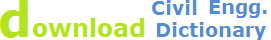degree of curve, degree of circular curve

Circular Curves in Engineering Survey

In the geometric design of motorways, railways, pipelines, etc., the design and setting out of curves is an important aspect of the engineer’s work. The initial design is usually based on a series of straight sections whose positions are defined largely by the topography of the area. The intersections of pairs of straights are then connected by horizontal curves.

In the vertical design, intersecting gradients are connected by curves in the vertical plane.

Circular curves are used to join intersecting straight lines (or tangents). Circular curves are assumed to be concave. Horizontal circular curves are used to transition the change in alignment at angle points in the tangent (straight) portions of alignments. An angle point is called a point of intersection or PI station; and, the change in alignment is defined by a deflection angle, Δ.

Types of Circular Curves are:

1. Simple Curve
2. Compound Curves
3. Broken Back Curves
4. Reverse Curves

A. Radius of a circular curveThe Radius is the distance from the center of the curve to any point on the circular curve.

B. Direction of a circular curve

The Direction of a Circular Curve is defined as the direction the curve tends, as stationing along the curve increases. Can be expressed as: Left, Right, North, East, South, West, free text

C. Central angle of a circular curve

The Central Angle of a Circular Curve is the angle at the center of radius of a circular arc included between the radii, passing through the beginning and ending of the arc.

D. Long Chord Length

The Long Chord Length is the straight line distance connecting the beginning of the curve and the end of the curve.

E. Degree of Curvature

The Degree of Curve is defined as the angle subtended by an arc whose length is 100 ft. A Radian is the angle subtended by an arc whose length equals the length of the Radius, or

57° 17’ 44.8” , or 57.295779513°.i. Curvature can be expressed in two ways, By:

1. Stating the length of the chord of the curve
2. Stating the radius of curvature

F. Laying out Circular Curves

1. Select tangents, and general curves making sure you meet minimum radius criteria

2. Select specific curve radii/spiral and calculate important points (see lab) using formula or table (those needed for design, plans, and lab requirements)

3. Station alignment (as curves are encountered)

4. Determine super and runoff for curves and put in table (see next lecture for def.)Site Map | Civil Engineering Training | Contact Us || © 2014 WebTechTix